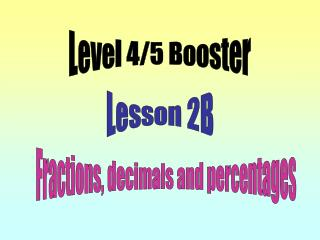DownloadDownload PresentationLesson 2B

# Lesson 2B

Download Presentation## Lesson 2B

- - - - - - - - - - - - - - - - - - - - - - - - - - - E N D - - - - - - - - - - - - - - - - - - - - - - - - - - -
##### Presentation Transcript

1. Level 4/5 Booster Lesson 2B Fractions, decimals and percentages

2. Objectives: • Use the equivalence of fractions, decimals and percentages to compare proportions. • Calculate percentages and fractions of an amount. Vocabulary: multiple recurring decimal Equivalent

3. T2.1B Find equivalent fractions. Write down any other sets of equivalent fractions.

4. T2.2B Give 50% of each number:

5. T2.2B Find 25% of each of the following numbers:

6. T2.2B Find ¾ of each of the following numbers.

7. How many times does 10% fit into 100%? There are 10 lots of 10% in 100%. What fraction is 10% the same as? 10% is the same as . How can you find 10% of a number? To find 10% we need to divide by 10. Explain how to find 10% of 450. 450 divided by 10 is 45 – move each digit one column to the right.

8. T2.3B Find 10% of each of the following amounts:

9. If I can find 10% what other percentages can I find? To find 20% we can do 2 x 10% To find 30% we can do………… To find 40% we can do ……………. How would you find 50%? Which is the easiest way to find 50%?

10. W/S 2.1B Find 10% and then use it to find other percentage amounts. 50% is 10% is 460 20% is 30% is On a copy of this spider diagram fill in the answers to those percentages stated and find some other percentages of your own choice.

11. Percentage Spider Diagram W/S2.1B On a copy of this diagram start with a number of your own choice and complete a percentage spider diagram.

12. Objectives: • Use the equivalence of fractions, decimals and percentages to compare proportions. • Calculate percentages and fractions of an amount. Vocabulary: multiple recurring decimal Equivalent

13. Thank you for your attention